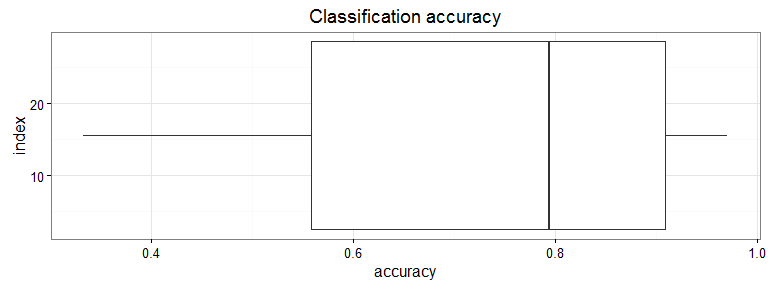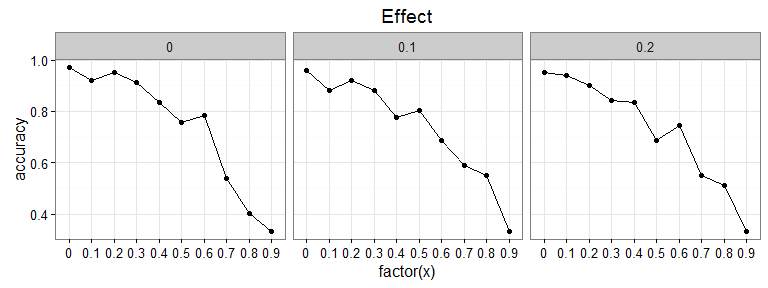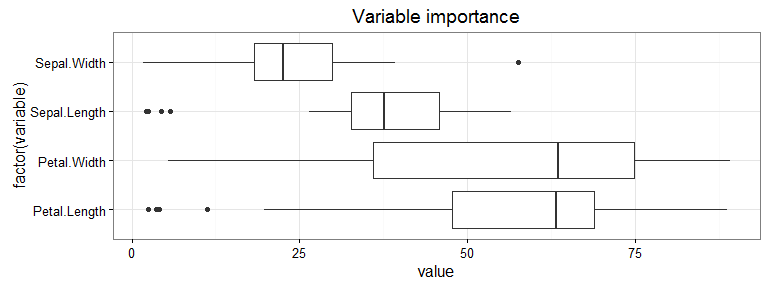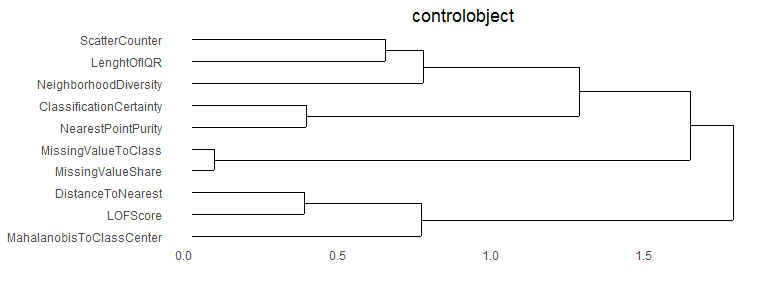# Preprosim

#### 2016-07-26

Data quality simulation can be used to check the robustness of data analysis findings and learn about the impact of data quality contaminations on classification. This package helps to add contaminations (noise, missing values, outliers, low variance, irrelevant features, class swap (inconsistency), class imbalance and decrease in data volume) to data and then evaluate the simulated data sets for classification accuracy. As a lightweight solution simulation runs can be set up with no or minimal up-front effort.

## Quick start

### Example 1: Creating contaminations

The package can be used to create contaminated data sets. Preprosimrun() is the main execution function and its default settings create 6561 contaminated data sets. In the example below argument ‘fitmodels’ is set to FALSE (not to compute classification accuracies) and default setup is used (argument ‘param’ is not given).

library(preprosim)
res <- preprosimrun(iris, fitmodels=FALSE)

All contaminated data sets can be acquired as a list from the data slot:

datasets <- res@data
length(datasets)
##  6561

The data set corresponding to a specific combination of contaminations can be acquired as a dataframe with getpreprosimdf() function.

df <- getpreprosimdf(res, c(0.1,0.1,0.1,0.1,0.1,0.1,0.1,0.1))
head(df)
##   x.Sepal.Length x.Sepal.Width x.Petal.Length x.Petal.Width         y
## 1       5.104000      3.498356       1.375905     0.2157918    setosa
## 2             NA      3.089296       1.428346     0.1626930 virginica
## 4       4.289669      3.202021       1.590556     4.2615823    setosa
## 5       5.193853      3.517478       1.441303     0.2102551    setosa
## 7       4.669934      3.336195       1.339044     0.2796277    setosa
## 8       5.108899      3.327911       1.449244     0.1741521    setosa

The second argument above has the contamination parameters in the following order:

str(res@grid, give.attr=FALSE)
## 'data.frame':    6561 obs. of  8 variables:
##  $noise : num 0 0.1 0.2 0 0.1 0.2 0 0.1 0.2 0 ... ##$ lowvar        : num  0 0 0 0.1 0.1 0.1 0.2 0.2 0.2 0 ...
##  $outlier : num 0 0 0 0 0 0 0 0 0 0.1 ... ##$ irfeature     : num  0 0 0 0 0 0 0 0 0 0 ...
##  $classswap : num 0 0 0 0 0 0 0 0 0 0 ... ##$ classimbalance: num  0 0 0 0 0 0 0 0 0 0 ...
##  $volumedecrease: num 0 0 0 0 0 0 0 0 0 0 ... ##$ misval        : num  0 0 0 0 0 0 0 0 0 0 ...

### Example 2: Classification accuracy of contaminated data sets

Preprosimrun() function with default value fitmodels=TRUE can be used to fit models and compute classification accuracy for each contaminated data set. Note that the selected model must be able to deal with missing values AND have an in-build variable importance scoring. Only ‘rpart’ and ‘gbm’ models are tested.

Parameter object is controlling, which contaminations are applied. In the example below the impact of missing values (primary, 10 contamination levels) and noise (secondary, 3 contamination levels ) on classification accuracy is studied. Classifier ‘rpart’ is used as a model instead of default ‘gbm’ and two times repreated holdout rounds are used. Argument ‘cores’ is not given, using 1 core by default.

res <- preprosimrun(iris, param=newparam(iris, "custom", x="misval", z="noise"), caretmodel="rpart", holdoutrounds = 2, verbose=FALSE)
preprosimplot(res)Specific dependencies between contaminations can be plotted by giving ‘xz’ argument to preprosimplot() function.

preprosimplot(res, "xz", x="misval", z="noise")The corresponding result data can be acquired with getpreprosimdata() function. In the exampe below ‘x’ and ‘y’ in str() function output correspond to arguments given in preprosimplot() and no other contaminations are applied similar to design of experiment (all other parameter values set to 0 zero).

data <- getpreprosimdata(res, "xz", x="misval", z="noise")
str(data)
## 'data.frame':    30 obs. of  9 variables:
##  $z : num 0 0.1 0.2 0 0.1 0.2 0 0.1 0.2 0 ... ##$ grid.lowvar        : num  0 0 0 0 0 0 0 0 0 0 ...
##  $grid.outlier : num 0 0 0 0 0 0 0 0 0 0 ... ##$ grid.irfeature     : num  0 0 0 0 0 0 0 0 0 0 ...
##  $grid.classswap : num 0 0 0 0 0 0 0 0 0 0 ... ##$ grid.classimbalance: num  0 0 0 0 0 0 0 0 0 0 ...
##  $grid.volumedecrease: num 0 0 0 0 0 0 0 0 0 0 ... ##$ x                  : num  0 0 0 0.1 0.1 0.1 0.2 0.2 0.2 0.3 ...
##  \$ accuracy           : num  0.971 0.961 0.951 0.922 0.882 ...

Variable importance (i.e. robustness of variables in classification task) in the contaminated data sets can be plotted:

preprosimplot(res, "varimportance")## Customization

The package includes eight build-in contaminations with parameters as contamination intensities. Contamination names, contents, parameter ranges and core definitions are presented below. For full definitions, please see the source code.

1. noise
• normal random number having original value in data as mean and parameter as standard deviation
• rnorm(length(x), x, param@noiseparam)
1. lowvar (low variance)
• parameter by which the original value is moved towards the mean of the variable
• 0 = none, 1=all values are mean
• multiplierdifftomean <- lowvarianceparameter * scale(x, scale=FALSE)
• newvalue <- x - multiplierdifftomean
1. misval (missing values)
• parameter for the share of missing values
• 0=none, 1 = all
• positionstomissingvalue <- sample(1:length(x), numberofmissingvalue)
• x[positionstomissingvalue] <- NA
1. irfeature (irrelevant features)
• parameter for the share of irrelevant features generated
• 0 = none, 1 = as many as there are variables in the original data
• numberofirrelevantfeatures <- as.integer(param@irfeatureparam * ncol(data@x))
• basedata <- data.frame(basedata, newvar=runif(nrow(data@x), -1, 1))
1. classswap (inconsistency)
• share of class labels that are swapped
• 0=none, 1=all
1. classimbalance
• share of observations to be removed from the most frequent class
• 0=none, 1=all
1. volumedecrease
• share of observations removed from the data
• 0=none, 1=all removed
• caret::createDataPartition(data@y, times = 1, p = param@volumedecreaseparam, list=FALSE)
1. outlier
• number of observations replaced with +IQR1.5 to +IQR2.0 outlier
• 0=none, 1=all
• outliers <- runif(d, smallestoutlier, largestoutlier)
• tobereplaced <- sample(1:length(x),d)
• x[tobereplaced] <- outliers

The package author is happy to include suggested new contaminations. Please contact the package author.

### Parameter structure

Each contamination has three sub parameters:

1. cols as columns the contamination is applied to
2. param as the parameter of the contamination itself (i.e. intensity of contamination)
3. order as order in which the parameter is applied to the data.

### Parameter construction

Parameter objects can be initialized with newparam constructor(). The constructor reads the data frame and sets the parameters. In the example below, first the parameters as set in a default manner, then as empty and lastly for a specific purpose.

pa <- newparam(iris)
pa1 <- newparam(iris, "empty")
pa2 <- newparam(iris, "custom", "misval", "noise")

### Parameter change

Parameters of an existing parameter object can be changed with changeparam() function.

pa <- changeparam(pa, "noise", "cols", value=1)
pa <- changeparam(pa, "noise", "param", value=c(0,0.1))
pa <- changeparam(pa, "noise", "order", value=1)

## Supporting packages

The data quality of a contaminated data set can be visualized with package preproviz. In the example below the data frame ‘df’ acquired above is visualized for data quality issue interdependencies.

library(preproviz)
viz <- preproviz(df)
plotVARCLUST(viz)In a similar manner the same data frame ‘df’ acquired above could be preprocesssed for optimal classification accuracy with package preprocomb.

library(preprocomb)
grid <- setgrid(preprodefault, df)
result <- preprocomb(grid)
result@bestclassification

For further information, please see package preproviz and preprocomb vignettes.